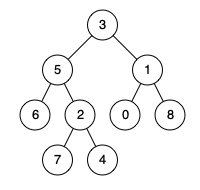# How to find lowest common ancestor of a binary tree ?

3.21K views
0

Given a binary tree, find the lowest common ancestor (LCA) of two given nodes in the tree.

According to the definition of LCA on Wikipedia: “The lowest common ancestor is defined between two nodes p and q as the lowest node in T that has both p and q as descendants (where we allow a node to be a descendant of itself).”

Given the following binary tree:  root = [3,5,1,6,2,0,8,null,null,7,4]Example 1:

Input: root = [3,5,1,6,2,0,8,null,null,7,4], p = 5, q = 1
Output: 3
Explanation: The LCA of nodes 5 and 1 is 3.

Example 2:

Input: root = [3,5,1,6,2,0,8,null,null,7,4], p = 5, q = 4
Output: 5
Explanation: The LCA of nodes 5 and 4 is 5, since a node can be a descendant of itself according to the LCA definition.

0

Below is a simple code in javascript with clean comments which are self explanatory.

/**
* Definition for a binary tree node.
* function TreeNode(val) {
*     this.val = val;
*     this.left = this.right = null;
* }
*/
/**
* @param {TreeNode} root
* @param {TreeNode} p
* @param {TreeNode} q
* @return {TreeNode}
*/
var lowestCommonAncestor = function(root, p, q) {
if (!root || root === p || root === q) return root  // if match is found or entire tree is traversed, return root
var left = lowestCommonAncestor(root.left, p, q);   // recursive tree traversal visit left node
var right = lowestCommonAncestor(root.right, p, q); // recursive tree traversal visit right node
if (!left)  return right;    // if not NULL return left node as p and q are in left subtree
if (!right) return left;   // if not NULL return right node as p and q are in left subtree
return root;   // return root node
};